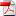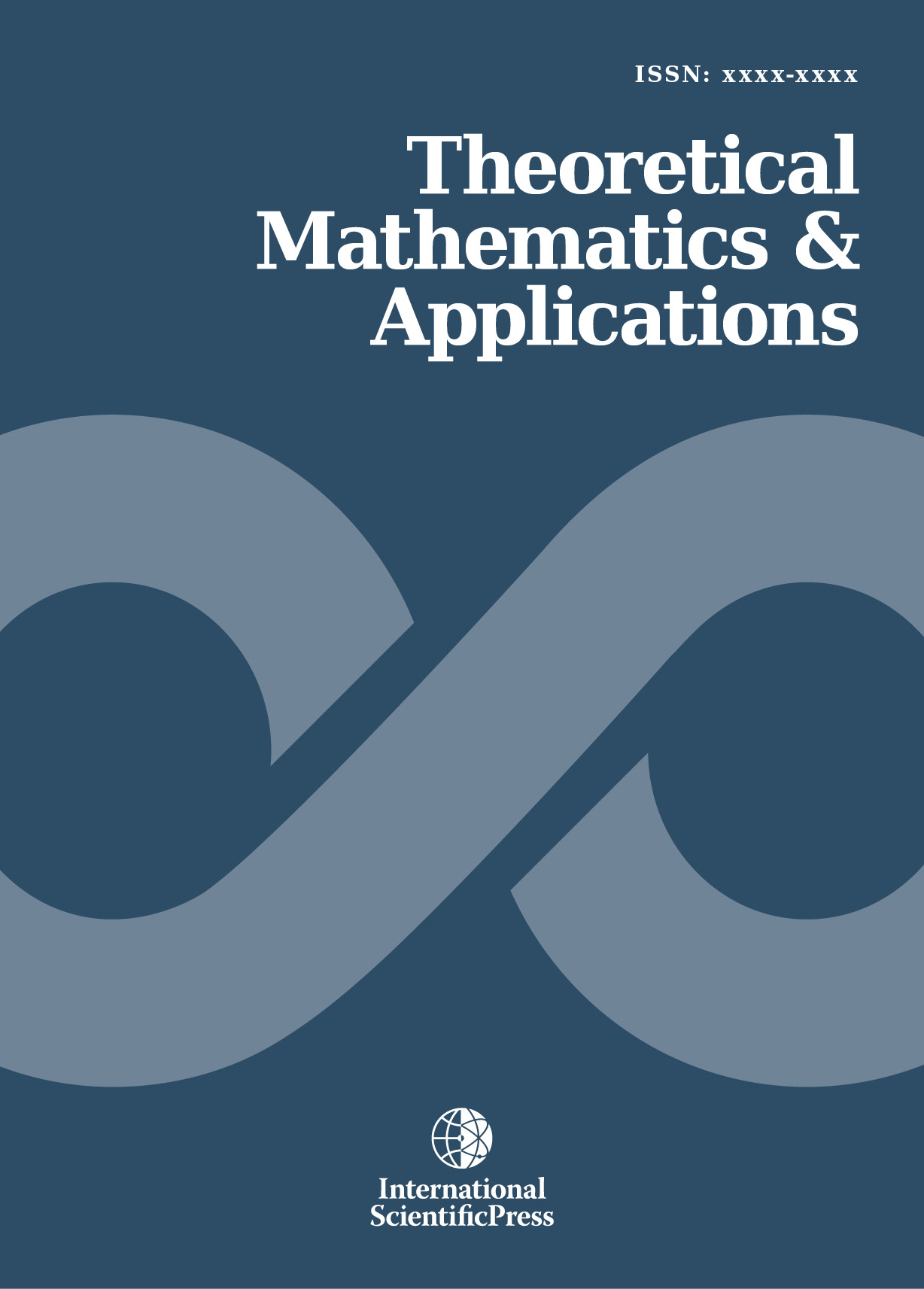# Theoretical Mathematics & Applications

#### A Fuzzy Minimum Cost Fuzzy Flow Problem with Fuzzy Time-Windows and Fuzzy Interval Bounds

•[ Download ]
• Abstract

In this paper, we present and describe a new version of the Minimum Cost Flow Problem (MCFP). This version is a Fuzzy Minimum Cost Fuzzy Flow Problem with Fuzzy Time-Windows and Fuzzy Interval Bounds (FMCFFPFTWFIB). The FMCFFPFTWFIB is a combinatorial optimization and an NP-hard problem. The FMCFFPFTWFIB of fuzzy interval data can be using two fuzzy minimum cost fuzzy flow of fuzzy time-windows problems with fuzzy crisp data. In this paper, the idea of Ghiyasvand was extended a fuzzy minimum cost fuzzy flow of fuzzy time-windows problem with fuzzy interval-valued lower, upper bounds and fuzzy flows. Also, this work is extended to the network with fuzzy lower, upper bounds and fuzzy flows. An application example network is given.

Mathematics Subject Classification: 90C27; 90C35; 68R10

Keywords: Optimization network, Minimum flow problem, Interval data, Time-windowsISSN: 1792-9687 (Print)
1792-9709 (Online)